• 向量自回归模型的原理和分析过程，包括其运用范围，原理讲解，应用实例模型
• 博主真是苦逼，最近定期要写研报，博主挑了个向量自回归模型（VAR）来研究，然而博主之前接触过的就只有MATLAB和python，matlab虽然做这种统计很方便，但是一个是博主好久不用啦有点生疏，还有一个是跟项目开发合在...
废话不多说，先开始分享：

20210127补充--------------------------------------

很多童鞋都问我要源代码和数据，本来是因为工作原因不想公开的，后来越来越多的人私信跟我反映说没有参照物实在太抽象了，那鉴于时间也比较久了，很多细节我也有点模糊了，一个一个解释不过来，因此，就发布一下原来我项目的源代码和当时用的数据，真的有需要的童鞋可以下载做参考。附下载地址：

------------------------------------------------------------------------------------------------------------------

1. 首先啥是VAR模型，我这里简略通俗的说一下，想看代码的童鞋直接跳到第3部分就好了：

以金融价格为例，传统的时间序列模型比如ARIMA,ARIMA-GARCH等，只分析价格自身的变化，模型的形式为：

其中称为自身的滞后项。

但是VAR模型除了分析自身滞后项的影响外，还分析其他相关因素的滞后项对未来值产生的影响，模型的形式为：

其中就是其他因子的滞后项。

总结一下，就是可以把VAR模型看做是集合多元线性回归的优点（可以加入多个因子）以及时间序列模型的优点（可以分析滞后项的影响）的综合模型。

VAR其实是一类模型，以上是最基础的VAR模型形式，其他还有SVAR,CVAR,VECM，同统称为VAR类模型。

2. VAR模型的建模步骤

这种数学模型都有固定的建模步骤：

1）画N个因子的序列相关图，计算相关系数 correlation coiffiant，查看一下线性相关度。（相关系数大小只反映线性相关程度，不反应非线性相关，如果等于0，不能排除存在非线性相关的可能。）

VAR模型要求所有因子数据同阶协整，也就是N个因子里面如果有一个因子数据不平稳，就要全体做差分，一直到平稳为止。

3）对应变量（yt）和影响因子（Xt）做协整检验

4）然后就是通过AIC,BIC,以及LR定阶。

一般来说是综合判断三者。AIC,BIC要最小的，比如-10的AIC就优于-1AIC，LR反之要最大的。但是具体偏重那个，就看个人偏好，一般来说，博主的经验是看AIC和LR，因为BIC的惩罚力度大于AIC，大多数时间不太好用。

具体的实现步骤一般是，把滞后项的阶数列一个范围，比如1-5，然后直接建模，其他啥都不看，先看AIC,BIC,LR的值。一般符合条件的不会只有一个，可以挑2-3个最好的，继续进行。

5）定阶完成后，就是估计参数，看参数的显著性。

好的模型所有参数的要通过显著性检验。

6）对参数进行稳定性检验

VAR除了对原始数据要进行平稳处理，估计出来的参数还需要检验参数稳定性。

这是为了查看模型在拟合时，数据样本有没有发生结构性变化。

有两张检验方法，这两种方法的基本概念是：

第一个是：AR根，VAR模型特征方程根的绝对值的倒数要在单位圆里面。

第二个是：cusum检验，模型残差累积和在一个区间内波动，不超出区间。

这里要注意的是CUSUM检验的原价设(H0)：系数平稳，备择假设才是不平稳。所以CUSUM结果要无法拒绝原假设才算通过。

只有通过参数稳定性检验的模型才具有预测能力,进行脉冲响应和方法分解分析才有意义。

7）使用乔里斯基正交化残差进行脉冲响应分析

举例：要分析和预测的是Y，影响Y的有两个因子X1，X2。

脉冲响应是1对1，根据以上条件，就要做两个脉冲响应分析,分别是：Y和X1，Y和X2。

看看不同因子上升或者下降，对Y的冲击的程度和方式（Y上升还是下降），以及持续时间。

8）使用乔里斯基正交化残差进行方差分解分析

举例：要分析和预测的是Y，影响Y的有两个因子X1，X2。

方差分解是1对1，根据以上条件，就要做两个方差分解分析,分别是：Y和X1，Y和X2。

9）为什么使用乔里斯基正交化残差？

因为进行方差分解和脉冲响应分析的时候，要求模型的残差为白噪声。但是！现实中，我们很难把所有影响Y的因素都囊括进方程，这就导致，现实中VAR模型的残差一般都不是白噪声。因此使用乔里斯基正交化来处理模型的残差。

VAR建模的时候以上面的条件为例，其实模型估计参数时会给出三个3个方程(应变量各自不同）：

方程1：

方程2：

方程3：

方程1的残差序列：

方程2的残差序列：

方差3的残差序列：

三个方程的乔里斯基正交化的步骤就是：

正交1：

正交2：

正交3：

正交4：

正交5：

最后用正交4/正交5，得到的序列就是乔里斯基正交化残差了。

乔里斯基正交化之前要对方程的变量按重要性排序，更重要的放在分子上。

3. 然后就是如何使用PYTHON 实现VAR模型的建模了：

以上的步骤是不是很庞大，看着很麻烦？但是电脑都会一下子嗖嗖嗖处理好的。

1）导入模块

# 模型相关包
import statsmodels.api as sm
import statsmodels.stats.diagnostic
# 画图包
import matplotlib.pyplot as plt
# 其他包
import pandas as pd
import numpy as np



2）画序列相关图

fig = plt.figure(figsize=(12,8))
plt.plot(changeXAUUSD,'r',label='XAU USD')
plt.plot(shfeXAU,'g',label='SHFE XAU')
plt.title('Correlation: ' + str(correlation))
plt.grid(True)
plt.axis('tight')
plt.legend(loc=0)
plt.ylabel('Price')
plt.show()

这里的数据格式应该是DataFrame里面的series格式，不过dataframe应该也可以吧，没试过。

adfResult = sm.tsa.stattools.adfuller(data,maxlags)
output = pd.DataFrame(index=['Test Statistic Value', "p-value", "Lags Used", "Number of Observations Used",
"Critical Value(1%)", "Critical Value(5%)", "Critical Value(10%)"],
columns=['value'])
output['value']['Number of Observations Used'] = adfResult
output['value']['Critical Value(10%)'] = adfResult['10%']

4）协整检验

python里面的协整检验通过coint（）这个函数进行的，返回P-value值，越小，说明协整关系越强。

result = sm.tsa.stattools.coint(data1,data2)

5）模型估计+定阶

这里PYTHON真的很烦，python有两套var估计，一个是VARMAX，一个是VAR。我看了官方文档后，觉得估计参数和定阶还是用VARMAX最好，因为可以返回很多东西，尤其是summary()里面的统计结果特别详细，直接包含了AIC,BIC,HQIC。

这里要注意，PYTHON定阶没有LR这个指标，要看LR的童鞋只能用EVIEWS和R了。不过AIC，BIC也够用了。

这里插入的数据只能是DATAFRAME格式的，不然就报错。

给大家看一下数据构造吧：

lnDataDict = {'lnSHFEDiff':lnSHFEDiff,'lnXAUDiff':lnXAUDiff}
data = lnDataDictSeries[['lnSHFEDiff','lnXAUDiff']]

这里的fitMod和resid变量后面会用到哦~~

#建立对象，dataframe就是前面的data，varLagNum就是你自己定的滞后阶数
orgMod = sm.tsa.VARMAX(dataframe,order=(varLagNum,0),trend='nc',exog=None)
#估计：就是模型
fitMod = orgMod.fit(maxiter=1000,disp=False)
# 打印统计结果
print(fitMod.summary())
# 获得模型残差
resid = fitMod.resid
result = {'fitMod':fitMod,'resid':resid}

6）系数平稳检验：CUSUM检验

这里也注意，Python这里不像EVIEWS，python没有办法算AR根，弄不到AR根图，但是python可以进行cusum检验。返回3各变量，第2个是P-value值，记得我之前说的吗，cusum检验要无法拒绝原假设，也就是说P-value值要大于0.05

这里的resid就是前面模型的resid

# 原假设：无漂移（平稳），备择假设：有漂移（不平稳）
result = statsmodels.stats.diagnostic.breaks_cusumolsresid(resid)

7）脉冲响应图

orthogonalized=True代表使用乔里斯基正交，这里很奇葩，官方文档没有加plt.show(),但是博主亲身试验，一定要加，不然画不出来。terms代表周期数。

# orthogonalized=True，代表采用乔里斯基正交
ax = fitMod.impulse_responses(terms, orthogonalized=True).plot(figsize=(12, 8))
plt.show()

8）方差分解图

这里要注意：

VARMAX很怪，没有做方差分解的方法，但是VAR这个方法里面有。（python就是这么任性！）

所以这里就用VAR重新估计，然后直接使用fevd进行方差分解。

打印summary（）可以看到方差分解的具体结果，plot可以画图，要记得加plt.show()哦~~

这里的dataFrame就是前面的data噢~~

md = sm.tsa.VAR(dataFrame)
re = md.fit(2)
fevd = re.fevd(10)
# 打印出方差分解的结果
print(fevd.summary())
# 画图
fevd.plot(figsize=(12, 16))
plt.show()

以上就是今日份的分享~~然后博主要开始吐槽了！

博主真是苦逼，最近定期要写研报，博主挑了个向量自回归模型（VAR）来研究，然而博主之前接触过的就只有MATLAB和python，matlab虽然做这种统计很方便，但是一个是博主好久不用啦有点生疏，还有一个是跟项目开发合在一起的话不方便。

然后博主现在天天用python，所以为了赶稿子，也只能硬着头皮用python搞一波了。但是，博主发现，全网，基本没有人用python搞过这种高级计量经济学模型，因为连范文都找不到1篇！！！！博主亲身尝试摸索后，虽然完成了研报，但是！博主还是要说，统计类的东西，要么用R，要么用EVIEWS，用Python真心苦逼！！！

主要是各个函数都藏在不知道什么旮旯角落里！！！有些还没有！比如AR根和AR图，要不是靠参考链接里R语言的一篇样板文，我都不知道还有个检验系数稳定性的方法叫cusum，再从另一篇样例里面找到python做cusum的样例。cusum的原假设和备择假设还跟普通检验不一样，反过来的，我查了好多中外文献，才确定这一点。python里面还没有EG因果关系检验，而协整关系检验的名字竟然叫coint……也是从另一篇样例文中找到的！！！都是泪…………

然后，python只能对VAR模型，VECM模型进行估计，其他var类模型，各位别白废力气寻找了，直接用EVIEWS或者R吧。

好啦~吐槽结束，博主可以保证，这是全网唯一的，最完整的利用python进行VAR模型建模的教程文了~~~

参考文献：

1. VARMAX官方样例

http://www.statsmodels.org/stable/examples/notebooks/generated/statespace_varmax.html

2. VARMAX官方文档

http://www.statsmodels.org/stable/generated/statsmodels.tsa.statespace.varmax.VARMAX.html?highlight=varmax

3.VARMAX fit官方文档

http://www.statsmodels.org/stable/generated/statsmodels.tsa.statespace.varmax.VARMAX.fit.html#statsmodels.tsa.statespace.varmax.VARMAX.fit

4.VARMAX fit返回值

http://www.statsmodels.org/stable/generated/statsmodels.tsa.statespace.mlemodel.MLEResults.html#statsmodels.tsa.statespace.mlemodel.MLEResults

5. CUSUM检验文献：平稳过程趋势项变点的CUSUM检验

https://www.ixueshu.com/document/1d642b472b5dc0717d721b29bfac1625.html#pdfpreview

6. CUSUM检验文献：关于CUSUM检验的改进

https://wenku.baidu.com/view/65d91ee1172ded630b1cb62c.html

7. cusum外文文献

https://doc.docsou.com/b77f40843604bd6fcc70f6d0b-10.html

8.python实现时间序列

https://max.book118.com/html/2017/1006/136205976.shtm

9.VAR python W3Cschool样例

https://www.w3cschool.cn/doc_statsmodels/statsmodels-examples-notebooks-generated-interactions_anova.html?lang=en

10.R语言实现VAR模型

https://blog.csdn.net/Imliao/article/details/80352158

11. python statsmodel手册

https://blog.csdn.net/qq_41518277/article/details/85101141#VARVAR_processes_175

12. python cusum检验方法说明：breaks_cusumolsresid

https://www.cherylgood.cn/doc/statsmodels/statsmodels-statistics/5bacda5744e2a52489c5292a.html

13.python 进行cusum检验样例

https://blog.csdn.net/CoderPai/article/details/83657386


展开全文python VAR
• ## VAR（向量自回归）模型

万次阅读 多人点赞 2020-06-14 10:03:34
VAR（向量自回归）模型是基于数据统计性质建立起来的模型，它把系统中的每个内生变量作为系统里所有其它内生变量滞后值的函数进行构建模型，从而把单变量的自回归模型推广到了多元时间序列组成的向量自回归模型。...
文章目录一、简介1.1 内生变量与外生变量1.2 VAR模型概念1.3 VAR模型结构1.4 VAR模型的特点二、模型的定阶（滞后阶数检验）三、模型的系数估计四、单位根检验五、格兰杰因果检验六、脉冲响应分析七、方差分解
一、简介
1.1 内生变量与外生变量
内生变量

内生变量是具有某种概率分布的随机变量，它的参数是联立方程系统估计的元素，是由模型系统决定的，同时也对模型系统产生影响。
内生变量–般都是明确经济意义变量。
一般情况下，内生变量与随机项相关，即 $Cov\left( Y_i,\varepsilon _i \right) \ne 0$
在联立方程模型中，内生变量既作为被解释变量，又可以在不同的方程中作为解释变量。

外生变量

外生变量一般是确定性变量，或者是具有临界概率分布的随机变量，其参数不是模型系统研究的元素。
外生变量影响系统，但本身不受系统的影响。
外生变量一般是经济变量、政策变量、虚拟变量。
一般情况下，外生变量与随机项不相关。

注意：一个变量是内生变量还是外生变量，由经济理论和经济意义决定，不是从数学形式决定。
1.2 VAR模型概念
向量自回归模型，简称VAR模型，是AR 模型的推广，是一种常用的计量经济模型。在一定的条件下，多元MA和ARMA模型也可转化成VAR模型。
VAR模型是用模型中所有当期变量对所有变量的若干滞后变量进行回归。
即
向量自回归模型把系统中每-一个内生变量作为系统中所有内生变量的滞后值的函数来构造模型，从而实现了将单变量自回归模型推广到由多元时间序列变量组成的“向量”自回归模型。
VAR模型常用于预测相互联系的时间序列系统以及分析随机扰动对变量系统的动态影响，主要应用于宏观经济学。是处理多个相关经济指标的分析与预测中最容易操作的模型之一。
由于向量自回归模型把每个内生变量作为系统中所有内生变量滞后值的函数来构造模型，从而避开了结构建模方法中需要对系统每个内生变量关于所有内生变量滞后值的建模问题。
1.3 VAR模型结构
单变量的时间序列的分析模式可以推广到多变量时间序列，建立向量自回归模型。向量自回归模型通常用于描述多变量时间序列之间的变动关系，不需要经济理论作为基础，从数据出发建立模型，是一种非结构化的模型。
非限制性向量自回归模型的一般表达式如下：
模型的基本形式是弱平稳过程的自回归表达式，描述的是在同一样本期间内的若干变量可以作为它们过去值的线性函数。
$Y_t=\varPhi _0+\varPhi _1Y_{t-1}+\cdots +\varPhi _pY_{t-p}+BX_t+\varepsilon _t\ \text{，\ }t=1,2,\cdots ,T$
其中
$Y_t=\left( \begin{array}{c} y_{1t}\\ y_{2t}\\ \vdots\\ y_{kt}\\ \end{array} \right) \text{，}\varepsilon _t=\left( \begin{array}{c} \varepsilon _{1t}\\ \varepsilon _{2t}\\ \vdots\\ \varepsilon _{kt}\\ \end{array} \right) \text{，}\varPhi _0=\left( \begin{array}{c} \phi _{10}\\ \phi _{20}\\ \vdots\\ \phi _{k0}\\ \end{array} \right)$
$\varPhi _i=\left( \begin{matrix} \phi _{11}\left( i \right)& \phi _{12}\left( i \right)& \cdots& \phi _{1k}\left( i \right)\\ \phi _{21}\left( i \right)& \phi _{22}\left( i \right)& \cdots& \phi _{2k}\left( i \right)\\ \vdots& \vdots& \ddots& \vdots\\ \phi _{k1}\left( i \right)& \phi _{k2}\left( i \right)& \cdots& \phi _{kk}\left( i \right)\\ \end{matrix} \right) \,\,\text{，\,\,}i=1,2,\cdots ,p$

$Y_t$ 表示 k 维内生变量列向量
$Y_{t-i}\text{，}i=1,2,\cdots ,p$ 为滞后的内生变量
$X_t$ 表示 d 维外生变量列向量，它可以是常数变量、线性趋势项或者其他非随机变量
p 是滞后阶数
T 为样本数目
$\varPhi _i$ 即 $\varPhi _1,\varPhi _2\cdots ,\varPhi _p$ 为 $k\times k$ 维的待估矩阵
B 为 $k\times d$ 维的待估矩阵
$\varepsilon _t\sim N\left( 0,\varSigma \right)$ 为 k 维白噪声向量，它们相互之间可以同期相关，但不与自己的滞后项相关（诸 $\varepsilon _t$ 独立同分布，而 $\varepsilon _t$ 中的分量不要求相互独立），也不与上式中右边的变量相关。$\varSigma$ 是 $\varepsilon _t$  的协方差矩阵， 是一个  $k\times k$ 的正定矩阵。.

比如 1 维 p 阶 向量自回归模型
$\left\{ \begin{array}{l} y_{1t}=\phi _{10}+\phi _{11}\left( 1 \right) y_{1,t-1}+\phi _{12}\left( 1 \right) y_{2,t-1}+\cdots +\phi _{1n}\left( 1 \right) y_{n,t-1}\\ \ \ \ \ \ \ \ \ +\phi _{11}\left( 2 \right) y_{1,t-2}+\phi _{12}\left( 2 \right) y_{2,t-2}+\cdots +\phi _{1n}\left( 2 \right) y_{n,t-2}\\ \ \ \ \ \ \ \ \ +\cdots\\ \ \ \ \ \ \ \ \ +\phi _{11}\left( p \right) y_{1,t-p}+\phi _{12}\left( p \right) y_{2,t-p}+\cdots +\phi _{1n}\left( p \right) y_{n,t-p}+\varepsilon _t\\ \end{array} \right.$
不含常数项或线性趋势项的向量自回归模型表达式为：
$Y_t=\varPhi _1Y_{t-1}+\cdots +\varPhi _pY_{t-p}+\varepsilon _t\ \text{，\ }t=1,2,\cdots ,T$
1.4 VAR模型的特点

不以严格的经济理论为依据。在建模过程中只需明确两件事：①共有哪些变量是相互有关系的，把有关系的变量包括在VAR模型中；②确定滞后期 p。使模型能反映出变量间相互影响的绝大部分。
VAR模型对参数不施加零约束。（对无显着性的参数估计值并不从模型中剔除，不分析回归参数的经济意义。）
VAR模型的解释变量中不包括任何当期变量，所有与联立方程模型有关的问题在VAR模型中都不存在（主要是参数估计量的非一致性问题）。
VAR模型的另一个特点是有相当多的参数需要估计。比如一个VAR模型含有三个变量，最大滞后期 p=3，则有27个参数需要估计。当样本容量较小时，多数参数的估计量误差较大。
无约束VAR模型的应用之一是预测。由于在VAR模型中每个方程的右侧都不含有当期变量，这种模型用于样本外一期预测的优点是不必对解释变量在预测期内的取值做任何预测。
用VAR模型做样本外近期预测非常准确。做样本外长期预测时，则只能预测出变动的趋势，而对短期波动预测不理想。

二、模型的定阶（滞后阶数检验）
滞后阶数检验需要考虑两个问题：

第一，如果滞后阶数 p 比较小，那么随机误差项会出现自相关的问题；
第二，在实际应用中，通常希望滞后阶数 p 足够大，进而能够更好的体现所构造的模型的动态特征，但是如果滞后阶数 p 过大时，那么模型所需要估计的参数就越多，将存在自由度太小的问题，如果没有足够多的样本数量，就会造成所需要估计参数不能有效的计算出来。

所以，在做滞后阶数检验之前，需要把各种因素都考虑在内，这样才能保证检验结果是有效的。
有两种方法可以做滞后阶数检验：

第–种方法，分析各种准则，最后确定滞后阶数，AIC准则、SC准则、HQ准则、LogL准则、最终预测误差（FPE）；.
第二种方法，分析似然比（LR），这种方法不会出现第一-种方法的无效结果。

第一种方法被学者们用的最多。.第一种方法中的五个指标在各个阶数的估计值，选取五个检验准则最小值数量最多的阶数即为模型的滞后期数。
比如三、模型的系数估计
对于向量自回归模型系统中的每一个方程都可以采用OLS（最小二乘估计）方法进行估计，同时估计量具有一致性和无偏性。
一个 k 维 p 阶向量自回归模型中，各方程中所有解释变量的滞后期是相同的，都为滞后 p 期，因此共估计得到  $p\times k^2+k$ 个系数。
四、单位根检验
时间序列平稳性是指–组数列的统计值与时间无关，不会随时间推移而变化，它通常是以因果关系为基础的数学模型的假设条件。

如果时间序列 $y_t$ 是一组平稳序列，那么经过计算分析得到其均值  $E\left( y_t \right)$ 不随时间变化而变化，其方差 $Var\left( y_t \right)$ 也不受时间的影响。
如果时间序列  $y_t$  不是一组平稳序列，那么它的均值和方差都会受到时间t影响，随之改变。

在VAR模型中，必须保证时间序列稳定。如果不能保证时间序列稳定，那么会导致两种结果：
第一，向量自回归系数的估计值是负数，做完 t 检验后，得到的结果是无效的；
第二，两个独立变量的相关关系或者回归关系是假的，使得模型的结果无效。
（1）DF 检验
DF 检验只适用于一阶自回归过程的平稳性检验
在一阶自回归序列中，
$y_t=\phi _1y_{t-1}+\varepsilon _t\ ,\ \varepsilon _t\sim N\left( 0,\sigma _{\varepsilon}^2 \right)$
该序列的特征方程为：
$\lambda -\phi _1=0$
特征根为：
$\lambda =\phi _1$
当特征根在单位圆内时：$\left| \phi _1 \right|<1$，该序列平稳
当特征根在单位圆上或单位圆外时：$\left| \phi _1 \right|\ge 1$，该序列非平稳
所以可以通过检验特征根是在单位圆内还是在单位圆上（外），来检验序列的平稳性，这种检验就称为单位根检验。
由于现实生活中绝大多数序列都是非平稳序列，所以单位根检验的原假设定为：
$H_0:\text{序列\ }y_t\ \text{非平稳}\Leftrightarrow H_0:\left| \phi _1 \right|\ge 1$
相应的备择假设为：$H_1:\text{序列\ }y_t\ \text{平稳}\Leftrightarrow H_1:\left| \phi _1 \right|<1$
检验统计量为：$t\left( \phi _1 \right) =\frac{\hat{\phi}_1-\phi _1}{S\left( \hat{\phi}_1 \right)}$
拒绝原假设，认为序列 $y_t$ 显著平稳
因为 DF 检验只适用于1阶自回归过程的平稳性检验，但实际上绝大多数时间序列都不会是一个简单的AR(1)过程。为了使DF检验能适用于AR(  p )过程的平稳性检验，对其进行了一定的修正，得到增广DF检验（augmented Dickey-Fuller)，简记为ADF检验。
对任一 AR ( p ) 过程
$y_t=\phi _1y_{t-1}+\cdots +\phi _py_{t-p}+\varepsilon _t$
特征方程：
$\lambda ^p-\phi _1\lambda ^{p-1}-\phi _2\lambda ^{p-2}-\cdots -\phi _p=0$
如果该方程所有的特征根都在单位圆内，即$\left| \lambda _i \right|<1\text{，}i=1,2,\cdots ,p$
则序列 $y_t$ 平稳
如果有一个单位根存在，不妨设
$\lambda _1=1$
则序列 $y_t$ 非平稳，且自回归系数之和恰好等于1：
$\left\{ \begin{array}{l} \lambda ^p-\phi _1\lambda ^{p-1}-\cdots -\phi _p\lambda ^{p-p}=0\\ \\ \xRightarrow{\lambda =1}\\ \\ 1-\phi _1-\cdots -\phi _p=0\\ \\ \Rightarrow\\ \\ \phi _1+\phi _2+\cdots +\phi _p=1\\ \end{array} \right.$
因而，对于AR( p )过程，可以通过检验自回归系数之和是否等于1来考察该序列的平稳性.
将.上述推广到 VAR( p ) 模型中，如果特征方程
$\left| I_N\lambda ^p-\varPhi _1\lambda ^p-\varPhi _2\lambda ^p-\cdots -\varPhi _p \right|=0$
的所有特征根都落在单位圆内，即 $\left| \lambda _i \right|<1\text{，}\left( i=1,2,\cdots ,p \right)$，那么就说 VAR( p ) 模型是协方差稳定的。
引入延迟算子B，如果 VAR( p ) 模型满足
$\left| I_N-\varPhi _1B-\varPhi _2B^2-\cdots -\varPhi _pB^p \right|=0$
的所有根都在单位圆外，模型也是协方差稳定的。

对
$y_t=\phi _1y_{t-1}+\cdots +\phi _py_{t-p}+\varepsilon _t$
进行等价变换：
$y_t-y_{t-1}=\phi _1y_{t-1}+\cdots +\phi _py_{t-p}-y_{t-1}+\varepsilon _t$
整理得
$\triangledown y_t=\left( \phi _1+\cdots +\phi _{p-1} \right) y_{t-1}-\left( \phi _2+\cdots +\phi _p \right) \triangledown y_{t-1}-\cdots -\phi _p\triangledown y_{t-p+1}+\varepsilon _t$
简记为
$\triangledown y_t=\alpha y_{t-1}+\beta _1\triangledown y_{t-1}+\cdots +\beta _{p-1}\triangledown y_{t-p+1}+\varepsilon _t$
式中
$\left\{ \begin{array}{l} \alpha =\phi _1+\phi _2+\cdots \phi _p-1\\ \\ \beta _j=-\phi _{j+1}-\phi _{j+2}-\cdots -\phi _p\ \text{，\ }j=1,2,\cdots ,p-1\\ \end{array} \right.$
若序列 $y_t$ 平稳，则$\phi _1+\phi _2+\cdots \phi _p<1$等价于 α<0
若序列 $y_t$ 非平稳，则至少存在一个单位根，有
$\phi _1+\phi _2+\cdots \phi _p=1$
等价于 α=0
则AR( p )过程单位根检验的假设条件可以确定为：
$H_0:\alpha =0\left( \text{序列非平稳} \right) \longleftrightarrow H_1:\alpha <0\left( \text{序列平稳} \right)$
$\tau =\frac{\hat{\alpha}}{S\left( \hat{\alpha} \right)}$
$S\left( \hat{\alpha} \right)$ 为参数 α 的样本标准差
利用 EVIEWS 可以进行平稳性检验
五、格兰杰因果检验
在有些情况下，时间序列分析也会出现伪相关问题，也就是可以计算出较大的相关系数的变量实际上并不相关。
针对此问题，格兰杰因果检验由此而生。格兰杰因果检验用于检验时间序列之间是否存在相关关系，它是能否建立脉冲函数的前提。
在VAR模型中，格兰杰检验的因果关系不是通常所说的因果关系（并非真正汉语意义上的“因果关系”），而是说先发生的事情对后发生的事情有–定的影响，或者说某个变量是否可以用来提高对其他相关变量的预测能力。所以，“格兰杰因果关系的实质是一-种“预测”关系
其实质是考量一个变量的滞后量能否加入到其他变量的公式中。当一个变量确实受到其他变量的滞后量影响时，可以称这两个变量具有格兰杰因果关系。
格兰杰因果检采取以下方式验证是否是真正的相关关系：
（1）估计当前的Y值被Y本身滞后期取值所能解释的程度；
（2）检验加入X的滞后期后，Y的被解释程度是否提高；
（3）如果满足条件（2），则X是Y的格兰杰成因，此时X的滞后期系数具有统计显著性。
具体是通过在向量自回归模型系统中考察序列滞后项的系数是否全为零来进行检验。以一个2维p阶平稳向量自回归模型为例
$\left\{ \begin{array}{l} y_{1t}=\phi _{10}+\phi _{11}\left( 1 \right) y_{1,t-1}+\phi _{11}\left( 2 \right) y_{1,t-2}+\cdots +\phi _{11}\left( p \right) y_{1,t-p}\\ \ \ \ \ \ \ \ \ +\phi _{12}\left( 1 \right) y_{2,t-1}+\phi _{12}\left( 2 \right) y_{2,t-2}+\cdots +\phi _{12}\left( p \right) y_{2,t-p}+\varepsilon _t\\ \end{array} \right.$
$\left\{ \begin{array}{l} y_{2t}=\phi _{20}+\phi _{21}\left( 1 \right) y_{1,t-1}+\phi _{21}\left( 2 \right) y_{1,t-2}+\cdots +\phi _{21}\left( p \right) y_{1,t-p}\\ \ \ \ \ \ \ \ \ +\phi _{22}\left( 1 \right) y_{2,t-1}+\phi _{22}\left( 2 \right) y_{2,t-2}+\cdots +\phi _{22}\left( p \right) y_{2,t-p}+\varepsilon _t\\ \end{array} \right.$
可以写成
$\left| \begin{array}{l} \left[ \begin{array}{c} y_{1t}\\ y_{2t}\\ \end{array} \right] =\left[ \begin{array}{c} \phi _{10}\\ \phi _{20}\\ \end{array} \right] +\left[ \begin{matrix} \phi _{11}\left( 1 \right)& \phi _{12}\left( 1 \right)\\ \phi _{21}\left( 1 \right)& \phi _{22}\left( 1 \right)\\ \end{matrix} \right] \left[ \begin{array}{c} y_{1,t-1}\\ y_{2,t-1}\\ \end{array} \right] +\left[ \begin{matrix} \phi _{11}\left( 2 \right)& \phi _{12}\left( 2 \right)\\ \phi _{21}\left( 2 \right)& \phi _{22}\left( 2 \right)\\ \end{matrix} \right] \left[ \begin{array}{c} y_{1,t-2}\\ y_{2,t-2}\\ \end{array} \right]\\ \\ \ \ \ \ \ \ \ \ \ \ \ \ \ \ \ +\cdots +\left[ \begin{matrix} \phi _{11}\left( p \right)& \phi _{12}\left( p \right)\\ \phi _{21}\left( p \right)& \phi _{22}\left( p \right)\\ \end{matrix} \right] \left[ \begin{array}{c} y_{1,t-p}\\ y_{2,t-p}\\ \end{array} \right] +\left[ \begin{array}{c} \varepsilon _{1t}\\ \varepsilon _{2t}\\ \end{array} \right]\\ \end{array} \right.$
检验原假设：$H_0:\ y_{2t}\text{不是}y_{1t}\text{的格兰杰原因}$
则通过F检验来检验联合假设
$\phi _{12}\left( 1 \right) =\phi _{12}\left( 2 \right) =\cdots =\phi _{12}\left( p \right) =0$
若检验结果拒绝原假设，即拒绝 $y_{2t}$ 不是 $y_{1t}$ 的格兰杰原因，则通常称 $y_{2t}$ 是 $y_{1t}$ 的格兰杰原因。
由于格兰杰因果关系检验是在向量自回归模型的基础上进行的，因此向量自回归模型本身的合理性对格兰杰因果关系检验的结果也是非常重要的。例如，向量自回归模型本身应当具有恰当的滞后期。
六、脉冲响应分析
在VAR模型中，脉冲响应分析的作用是可以分析某个变量对另一个变量的影响时间和幅度。研究当扰动项发生变化时，对整个模型系统产生的影响，用来描述一个变量的变动怎样影响模型其他所有的变量。
如果时间序列是稳定的，虽然前几期受到外部冲击的影响，该变量会处于一个变化的状态，但经过一段时间，最终会处于-一个平稳的状态。
由于向量自回归模型表达式中所需要估计的参数非常多，并且一个系数只能反应局部关系。
也就是，VAR模型中的各个等式中的系数并不是研究者最终关注的对象，对模型表达式中的系数的研究意义并不大。但是如果考虑一个扰动项变动，或者受到一个干扰或冲击，各个变量之间的动态关系，也就是系统的动态反应，是具有–定意义的。
脉冲响应函数
在参数估计量的评价标准中，一般包含无偏性、有效性、相合性和一致性，而VAR模型参数的普通最小二乘法估计量只具有–致性，因此要解释复杂的经济问题，单个参数估计值是很难完成的。
一个有效的对VAR模型进行分析的方法就是脉冲响应函数。
脉冲响应函数研究的是随机干扰项遭受冲击后内生变量的反应，用来描述对随机干扰项施加一一个冲击后对内生变量的当期值和未来值造成的影响。
在实际的应用中，由于VAR模型是一种非理论性的模型，因此在对VAR模型的分析中，很少研究一个变量的变化对另-一个变量的影响，而是专注于当一个随机误差项变化时（对随机误差项施加冲击），对系统的动态影响。
七、方差分解
在VAR模型中，得到了某个变量对另一个变量的解释度后，能够分析出该变量的重要性。变量会产生一些随机误差项，这些随机误差项都包含着重要的信息，方差分解的结果能够把这些信息全部说明出来。方差分解的作用非常大，这个过程的作用是能够分析某个变量对另-一个变量的解释度。
如果说脉冲响应函数是来描述数学模型中的任一内生变量的正交冲击对其他变量造成的影响，那么方差分解就是分析各个内生变量的正交冲击对目标内生变量冲击的贡献比例，进而判断分析各个变量的重要性。
参考：
《浅析中国对美贸易额的影响因素》_张康琦
《基于VAR模型的GDP和CPI对居民生活水平的影响分析》_陈小龙
《向量自回归模型扰动分布的光滑检验及其应用》_钟嫕姝


展开全文• 详细讲解向量自回归的历史发展作用和实战应用，有着非常丰富的逻辑脉络和实战案例VAR
• 这次研究了一个问题，要用PVAR(面板向量自回归模型)，在网上找的教程基本上都是Stata或者Eviews的教程，而鲜有R实现PVAR的教程，这里总结分享一下我摸索的PVAR用R实现的整个过程。 ...
这次研究了一个问题，要用PVAR(面板向量自回归模型)，在网上找的教程基本上都是Stata或者Eviews的教程，而鲜有R实现PVAR的教程，这里总结分享一下我摸索的PVAR用R实现的整个过程。码字不易，喜欢请点赞！！！谢谢
使用R来实现PVAR用到的包是panelvar，panelvar的文档下载链接：
https://cran.r-project.org/web/packages/panelvar/panelvar.pdf

1.数据样式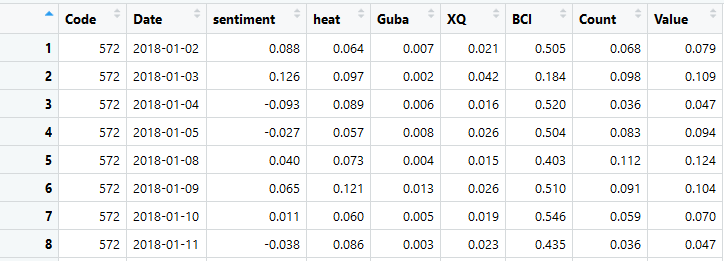其中Code和Date两列是面板时间序列索引。
2.使用plm包的pdata.frame将dataframe数据转成面板dataframe
library(plm)
##使用pdata.frame()函数将外部读取的数据转换为plm包可以识别的面板格式，
##使用index参数标记名为Code和Date的列，分别对应股票代码和时间
pdata=pdata.frame(data,index=c("Code","Date"))

3.面板数据平稳性检验
##使用plm包中的purtest()进行面板数据的平稳性检验。
##其中，参数object设定待检验变量；
##exo参数设定是否包含截距项(intercept)和趋势项(trend)；
##test参数定义面板平稳性检验的方法;
##lags参数定义信息准则，使用“AIC”和“SIC”进行定义；
##pmax参数定义最大滞后期；
purtest(object=pdata[,3],exo="intercept",test="ips",lags="AIC",pmax=10)

平稳性检验结果若p值小于0.05，则表示平稳
4.使用panelvar包的pvargmm函数进行GMM-PVAR分析以及确定最优滞后期
library(panelvar)
##data参数定义数据集；
##dependent_vars参数定义内生变量；
##exog_vars参数定义外生变量；
##lags参数定义滞后期；
##transformation参数定义GMM模型的类型，包括水平模型和差分模型(First-difference "fd" or forward orthogonal deviations "fod")；
##steps参数定义GMM模型的估计程序，包括一步估计、两步估计和多步估计；
##max_instr_dependent_vars以及 max_instr_predet_vars定义GMM模型工具变量的滞后期，我们按照面板GMM的常规设置，设为99期。
gmmlag = pvargmm(dependent_vars=c("sentiment","heat","Guba","XQ","BCI"),data=pdata,lags=5,exog_vars=c("Count","Value"),
transformation="fd",steps="twostep",max_instr_dependent_vars=99)

注：一般VAR和SVAR都是先确定最优滞后阶数然后再进行模型模拟，但是R使用panelvar包实现PVAR时，需要先使用模型模拟，然后使用Andrews_Lu_MMSC函数计算模型拟合结果的AIC、BIC、HQIC值，从而比较不同滞后阶数的准则值来得到最优滞后阶数。
Andrews_Lu_MMSC(gmmlag)

5.模型估计结果过度识别检验
##原假设：工具变量是外生的。
##若拒绝原假设，则说明存在工具变量与扰动项相关。
hansen_j_test(gmmlag)

6.稳定性检验
stability(gmmlag)

7.脉冲响应分析
##使用oirf()函数进行脉冲响应分析



展开全文R语言 PVAR
•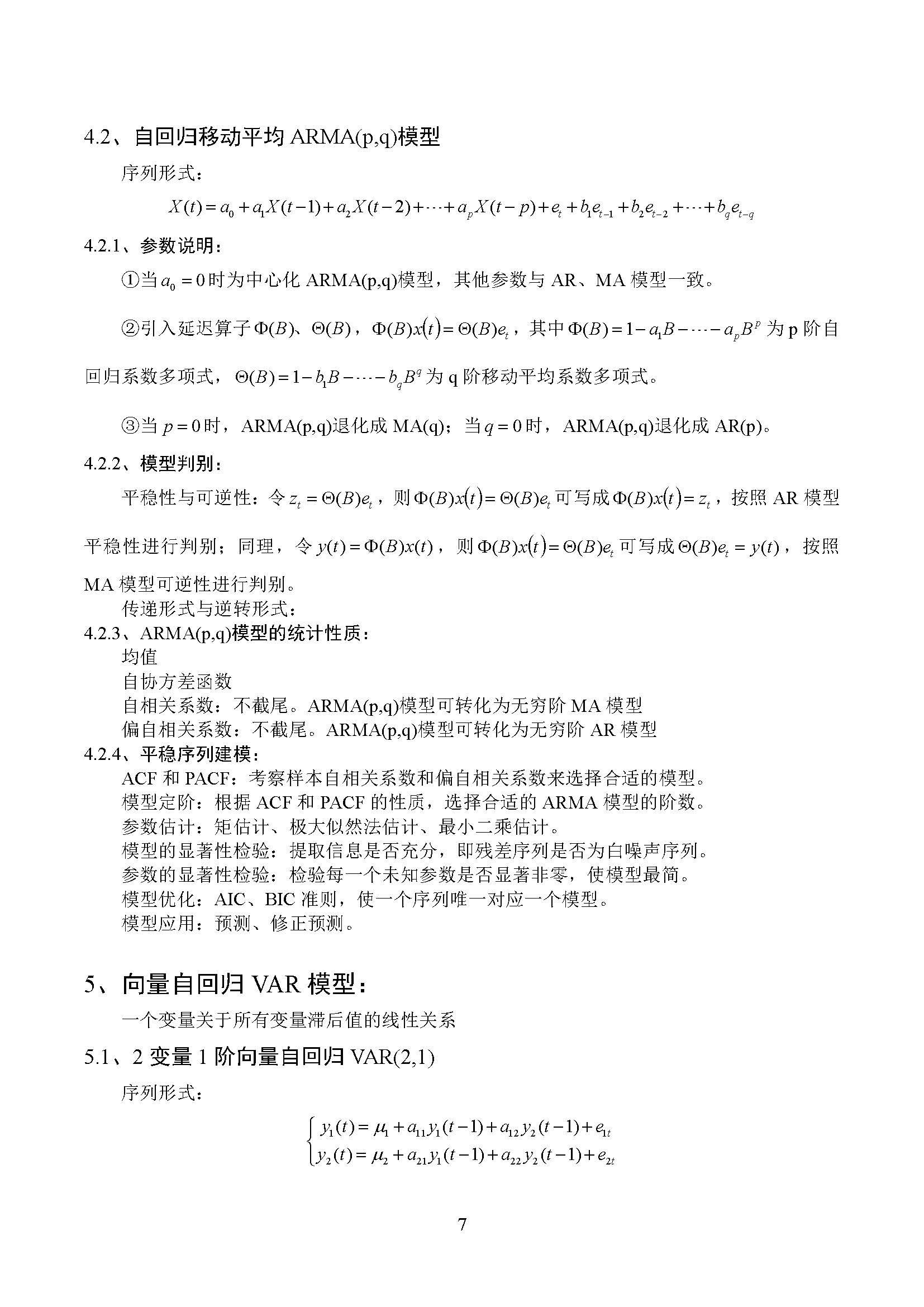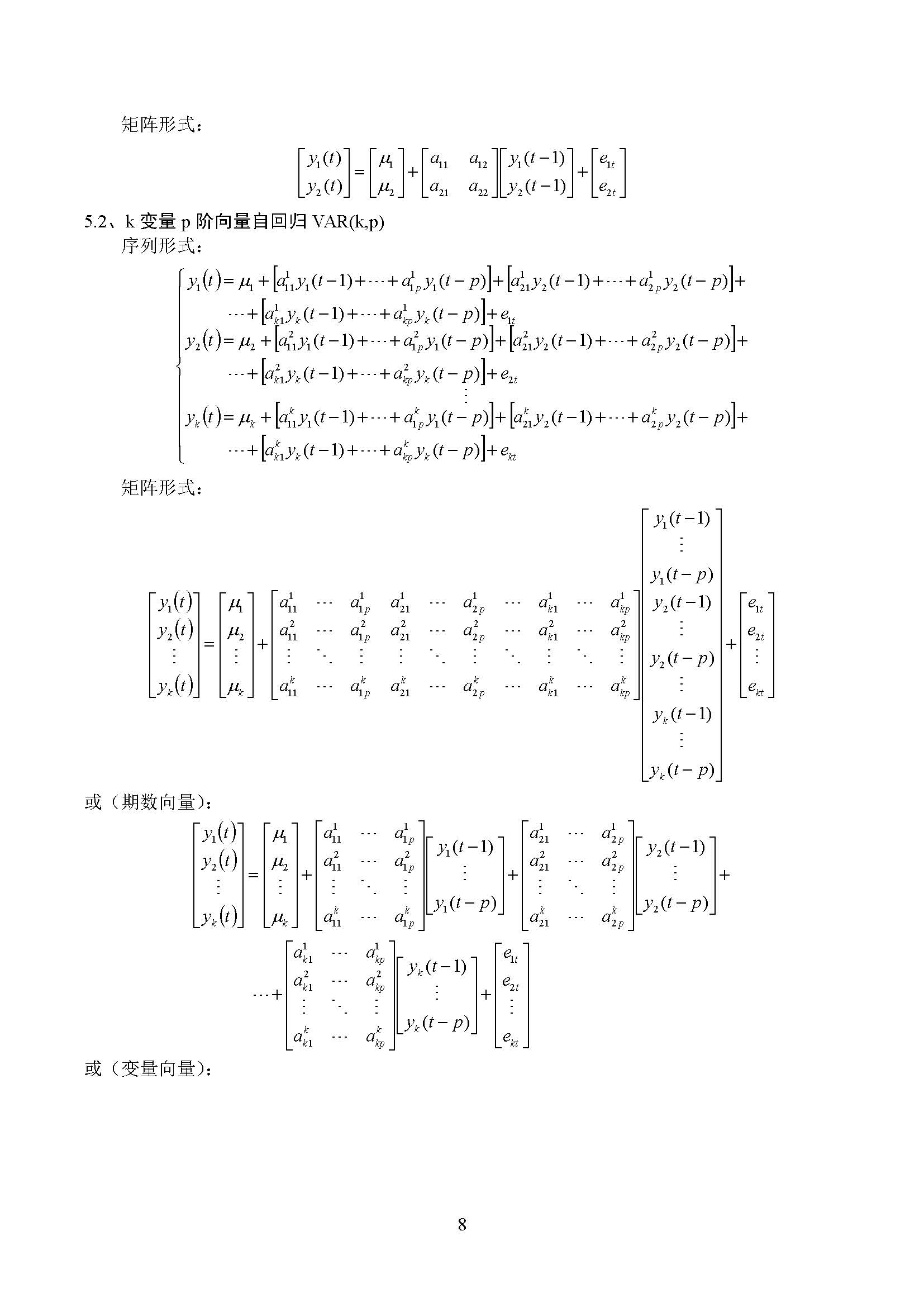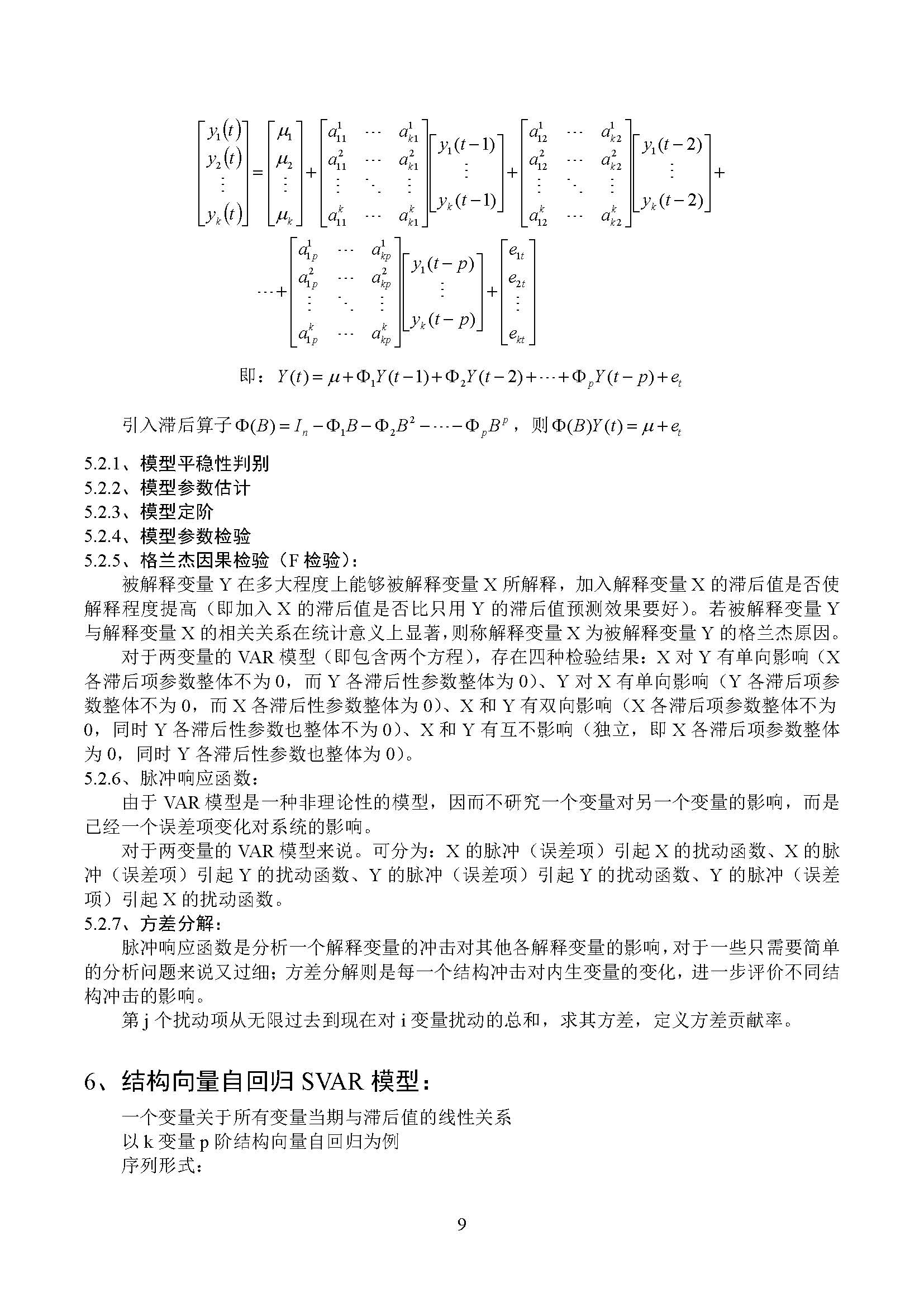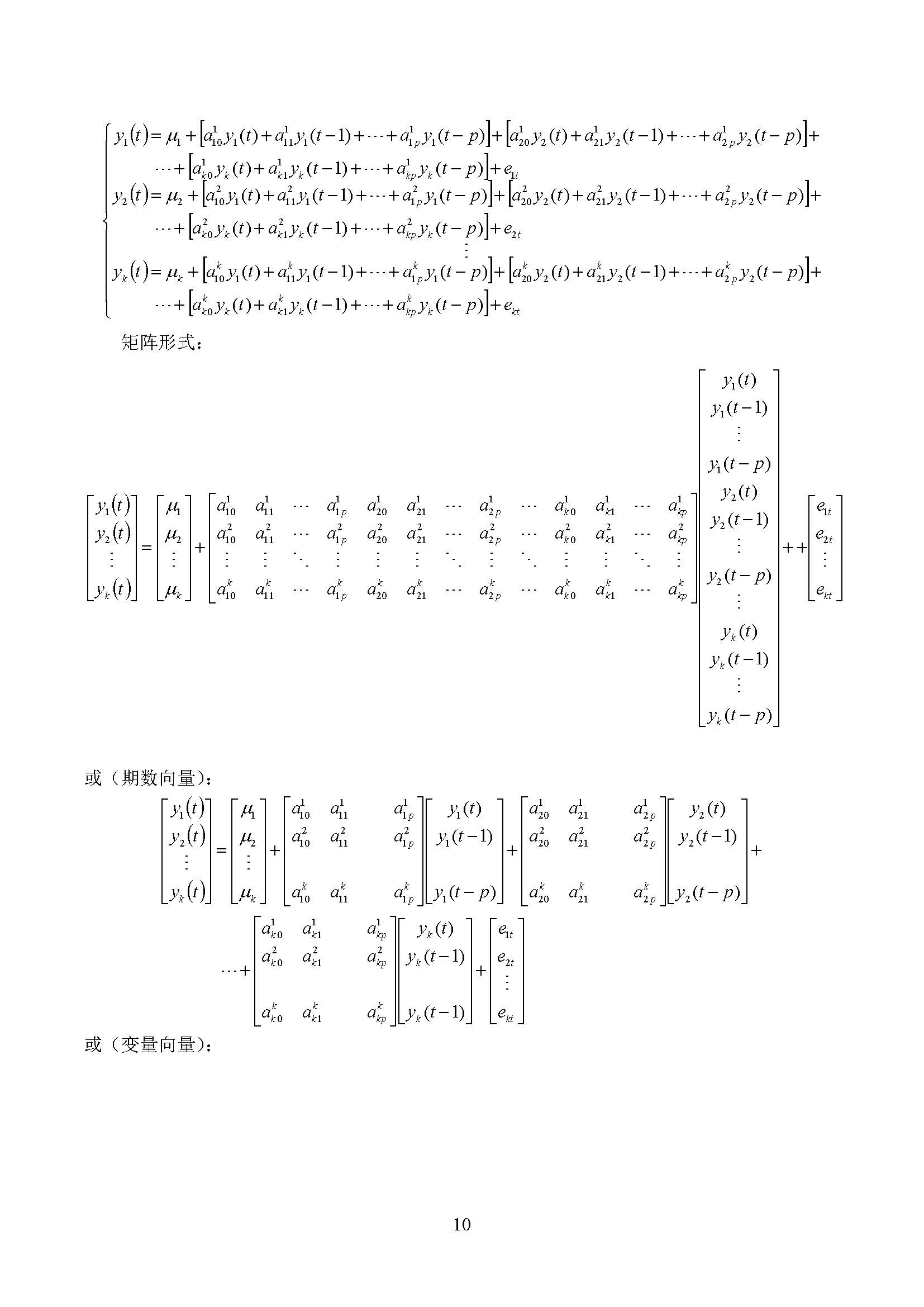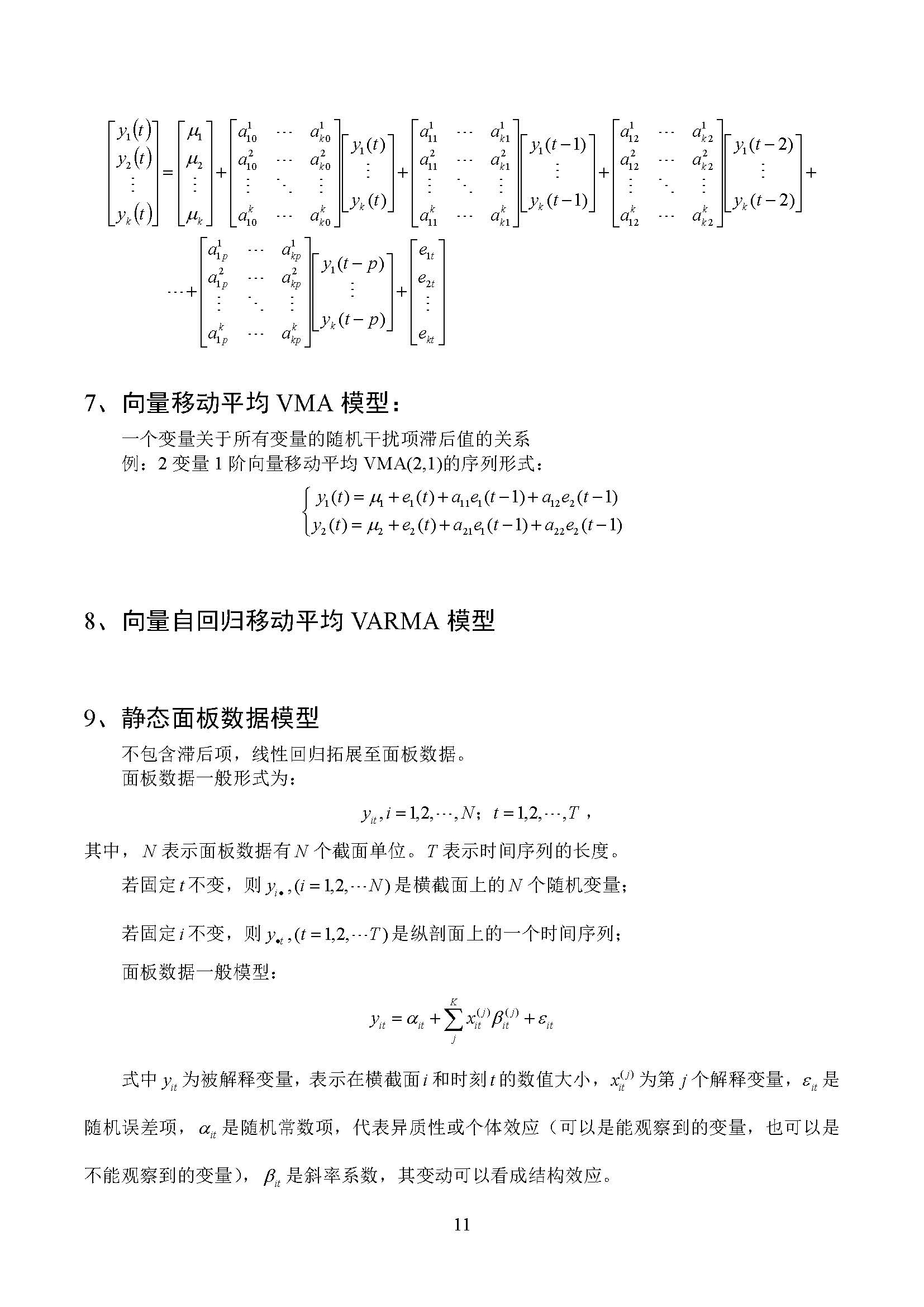展开全文线性代数 矩阵机器学习 数学建模 模型
• 向量自回归模型（简称VAR模型）是一种常用的计量经济模型，由克里斯托弗·西姆斯（Christopher Sims）提出。它是AR模型的推广。 ...
• 自从Sims（1980）发表开创性的论文以来，向量自回归模型已经成为宏观经济研究中的关键工具。这篇文章介绍了VAR分析的基本概念，并指导了简单模型的估算过程。 单变量自回归 VAR代表向量自回归。为了理解这意味着...R语言 VAR 脉冲响应
• 一、向量自回归检验 1. 向量自回归检验(VAR)：Vector autoregression 单独建一个AR模型，不足以说明每个变量的影响。此时就需要做VAR模型。 VAR模型针对的是平稳性序列，如果是非平稳性的 ，实质上则进行的是...
• 向量自回归模型VAR是计量经济学中的一个概念，用于多元时间序列相关关系的分析。详细概念我就不赘述了，具体的定义和技术流程可以看计量经济学的相应书籍、人大经济论坛的视频或者是公众号“财经节析”的介绍。这个...R VAR
•线性代数 矩阵
• VAR（向量自回归）模型的stata操作 毕设发现好多姐妹都在用VAR模型，可能这是金融孩纸们必备的模型吧。毕竟过于复杂的市场总是有乱七八糟的关系。so，整一个小栗子说明一下VAR模型和它的stata命令！希望大家和正在为...数据分析
• 政府发布了一揽子刺激计划，其中...使用r语言随机波动模型sv处理时间序列中的随机波动 7.r语言时间序列tar阈值自回归模型 8.r语言k-shape时间序列聚类方法对股票价格时间序列聚类 9.python3用arima模型进行时间序列预测R语言 VAR模型
• ## Stata 中的向量自回归模型(VAR)

万次阅读 多人点赞 2018-11-21 12:09:44
在单变量回归中，一个平稳的时间序列 yty_tyt​ 经常被模型化为 AR 过程： yt=α0+α1yt−1+α2yt−2+⋯+αkyt−k+ϵt y_t=\alpha_0+\alpha_1 y_{t-1}+\alpha_2 y_{t-2}+\dots +\alpha_k y_{t-k}+\epsilon_t yt​...
• 有限制的模型称为周期自回归模型，被称为 模型 其中 并且 这是一个VAR(1) 模型，因此 可以来估计这个模型 par(wts=tsq, type="PAR", p=1) > PAR(model) 特征方程为 所以有一个(季节性的)单位根，如果 但在这里显然...R语言 arima VAR 时间序列
• . matlist e(Sigma) | inflation unrate ffr -------------+--------------------------------- inflation | .1849129 unrate | -.0064425 .0636598 ffr | .0788766 -.09169 .6324shell
• 原文链接：...使用r语言随机波动模型sv处理时间序列中的随机波动 7.r语言时间序列tar阈值自回归模型 8.r语言k-shape时间序列聚类方法对股票价格时间序列聚类 9.python3用arima模型进行时间序列预测R语言...